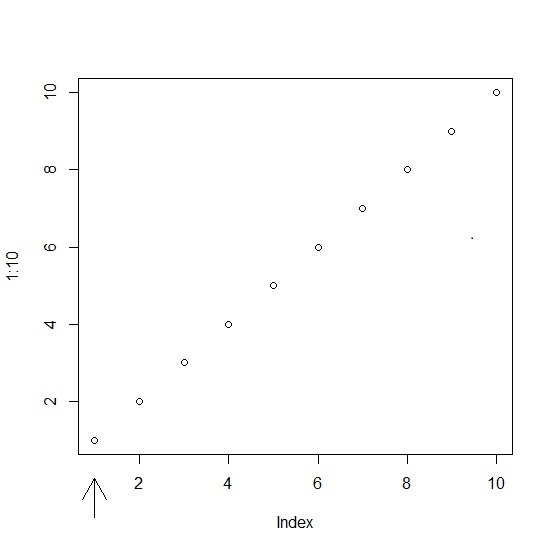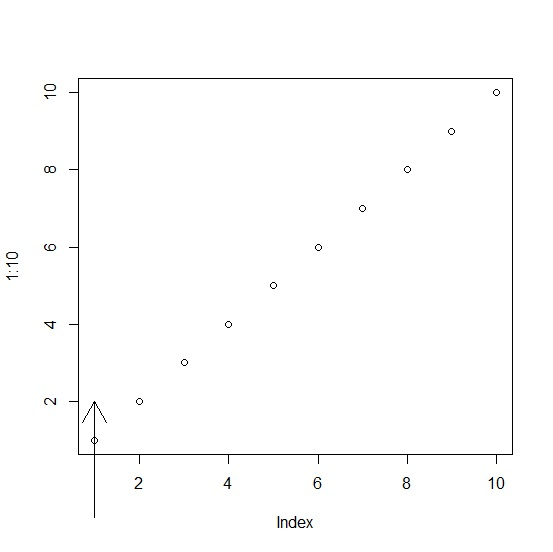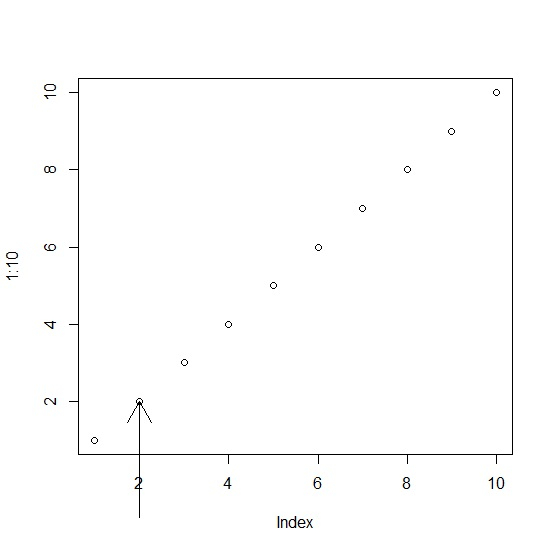# How to create a perpendicular arrow in base R plot?

To create a perpendicular arrow in base R plot, we can use arrows function. There are five arguments of arrows function that will be used to create the perpendicular arrow. The first four values define the position of the arrow and the last argument xpd allows R to create the arrow. Check out the below examples to understand how it works.

## Example

plot(1:10)
arrows(1,-1,1,0,xpd=TRUE)

## Output## Example

plot(1:10)
arrows(1,-1,1,2,xpd=TRUE)

## Output## Example

plot(1:10)
arrows(2,-1,2,2,xpd=TRUE)

## Output